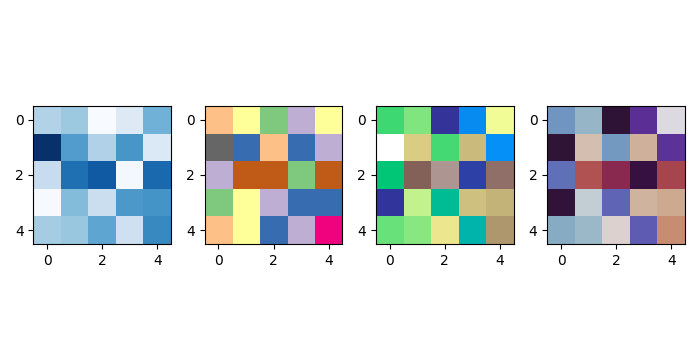# Drawing multiple figures in parallel in Python with Matplotlib

To draw multiple figures in parallel in Python with matplolib, we can take the following steps−

• Create random data using numpy.
• Add a subplot to the current figure, nrows=1, ncols=4 and at index=1.
• Display data as an image, i.e., on a 2D regular raster, using imshow() method with cmap="Blues_r".
• Add a subplot to the current figure, nrows=1, ncols=4 and at index=2.
• Display data as an image, i.e., on a 2D regular raster, using imshow() method with cmap="Accent_r".
• Add a subplot to the current figure, nrows=1, ncols=4 and at index=3.
• Display data as an image, i.e., on a 2D regular raster, using imshow() method with cmap="terrain_r"
• Add a subplot to the current figure, nrows=1, ncols=4 and at index=4.
• Display data as an image, i.e., on a 2D regular raster, using imshow() method with cmap="twilight_shifted_r".
• To display the figure, use show() method.

## Example

import numpy as np
from matplotlib import pyplot as plt
plt.rcParams["figure.figsize"] = [7.00, 3.50]
plt.rcParams["figure.autolayout"] = True
data = np.random.rand(5, 5)
plt.subplot(1, 4, 1)
plt.imshow(data, cmap="Blues_r")
plt.subplot(1, 4, 2)
plt.imshow(data, cmap="Accent_r")
plt.subplot(1, 4, 3)
plt.imshow(data, cmap="terrain_r")
plt.subplot(1, 4, 4)
plt.imshow(data, cmap="twilight_shifted_r")
plt.show()

## Output